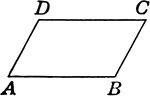### Parallelogram

Parallelogram with angles labeled.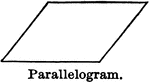### Parallelogram

Illustration of a parallelogram, a quadrilateral which has opposite sides parallel.### Parallelogram With Vertices Labeled

An illustration of a parallelogram with vertices labeled.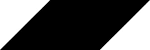### Parallelogram

Tangrams, invented by the Chinese, are used to develop geometric thinking and spatial sense. Seven figures…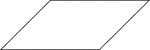### Parallelogram

Tangrams, invented by the Chinese, are used to develop geometric thinking and spatial sense. Seven figures…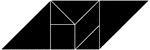### Parallelogram

Tangrams, invented by the Chinese, are used to develop geometric thinking and spatial sense. Seven figures…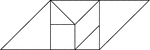### Parallelogram

Tangrams, invented by the Chinese, are used to develop geometric thinking and spatial sense. Seven figures…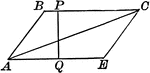### Altitude and Diagonal of a Parallelogram

Illustration of a parallelogram with altitude and diagonal drawn.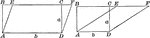### Area of a Parallelogram

Illustration of parallelogram AEFC drawn two different ways with base b and altitude/height a used to…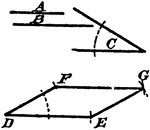### Construction Of A Parallelogram

An illustration showing the construction used to erect a parallelogram given two sides and an angle.…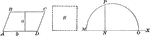### Parallelogram Used to Construct Equivalent Square

Illustration of a how to construct a square equivalent to a given parallelogram.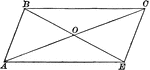### Parallelogram With Diagonals Bisecting Each Other

Illustration of a parallelogram with diagonals drawn to show they bisect each other.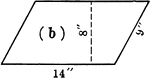### Parallelogram With Dimensions

Parallelogram with dimensions labeled. Parallelogram can be used to calculate area.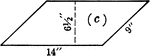### Parallelogram With Dimensions

Parallelogram with dimensions labeled. Parallelogram can be used to calculate area.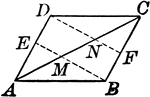### Parallelogram With Lines From opposite Vertices to Midpoints

Illustration to show that when lines from two opposite vertices of a parallelogram to the midpoints…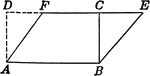### Parallelogram and Rectangle Relationship

Parallelogram with dimensions drawn to show relationship to the area of a rectangle.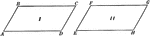### 2 Equal Parallelograms

Illustration used to prove the theorem "Two parallelograms are equal if two sides and the included angle…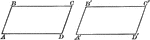### Equal Parallelograms

Illustration of a two equal parallelograms. Two parallelograms are equal, if two sides and the included…### Parrot Fish

Tangrams, invented by the Chinese, are used to develop geometric thinking and spatial sense. Seven figures…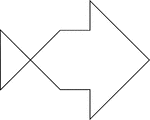### Parrot Fish

Tangrams, invented by the Chinese, are used to develop geometric thinking and spatial sense. Seven figures…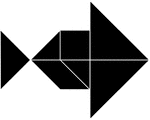### Parrot Fish

Tangrams, invented by the Chinese, are used to develop geometric thinking and spatial sense. Seven figures…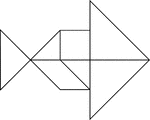### Parrot Fish

Tangrams, invented by the Chinese, are used to develop geometric thinking and spatial sense. Seven figures…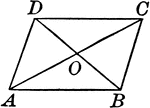Illustration to show that if the diagonals of a quadrilateral bisect each other, the figure is a parallelogram.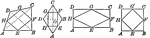### Quadrilaterals With Lines Joining Midpoints

Illustrations to show quadrilaterals with lines joining the midpoints of the sides of any quadrilateral,…### Rabbit Lieing Down

Tangrams, invented by the Chinese, are used to develop geometric thinking and spatial sense. Seven figures…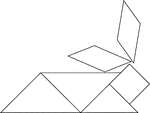### Rabbit Lieing Down

Tangrams, invented by the Chinese, are used to develop geometric thinking and spatial sense. Seven figures…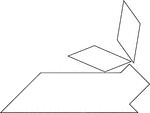### Rabbit Lying Down

Tangrams, invented by the Chinese, are used to develop geometric thinking and spatial sense. Seven figures…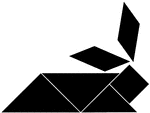### Rabbit Lying Down

Tangrams, invented by the Chinese, are used to develop geometric thinking and spatial sense. Seven figures…### Rabbit Siting on Its Hind Legs

Tangrams, invented by the Chinese, are used to develop geometric thinking and spatial sense. Seven figures…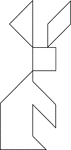### Rabbit Siting on Its Hind Legs

Tangrams, invented by the Chinese, are used to develop geometric thinking and spatial sense. Seven figures…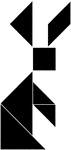### Rabbit Siting on Its Hind Legs

Tangrams, invented by the Chinese, are used to develop geometric thinking and spatial sense. Seven figures…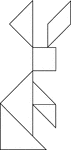### Rabbit Siting on Its Hind Legs

Tangrams, invented by the Chinese, are used to develop geometric thinking and spatial sense. Seven figures…### Reclining Man Facing Left

Tangrams, invented by the Chinese, are used to develop geometric thinking and spatial sense. Seven figures…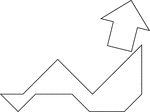### Reclining Man Facing Left

Tangrams, invented by the Chinese, are used to develop geometric thinking and spatial sense. Seven figures…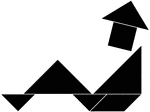### Reclining Man Facing Left

Tangrams, invented by the Chinese, are used to develop geometric thinking and spatial sense. Seven figures…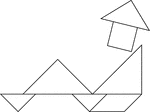### Reclining Man Facing Left

Tangrams, invented by the Chinese, are used to develop geometric thinking and spatial sense. Seven figures…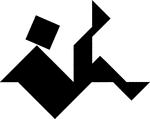### Reclining Man Facing Right

Tangrams, invented by the Chinese, are used to develop geometric thinking and spatial sense. Seven figures…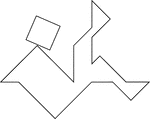### Reclining Man Facing Right

Tangrams, invented by the Chinese, are used to develop geometric thinking and spatial sense. Seven figures…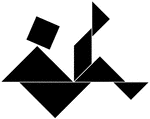### Reclining Man Facing Right

Tangrams, invented by the Chinese, are used to develop geometric thinking and spatial sense. Seven figures…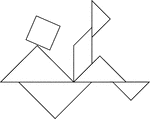### Reclining Man Facing Right

Tangrams, invented by the Chinese, are used to develop geometric thinking and spatial sense. Seven figures…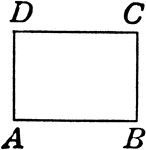### Rectangle

Rectangle with angles labeled. Parallelogram with right angles.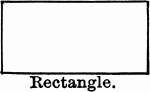### Rectangle

Illustration of a rectangle, which is a parallelogram with all angles right angles.### Rectangle

Tangrams, invented by the Chinese, are used to develop geometric thinking and spatial sense. Seven figures…### Rectangle

Tangrams, invented by the Chinese, are used to develop geometric thinking and spatial sense. Seven figures…### Rectangle

Tangrams, invented by the Chinese, are used to develop geometric thinking and spatial sense. Seven figures…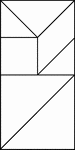### Rectangle

Tangrams, invented by the Chinese, are used to develop geometric thinking and spatial sense. Seven figures…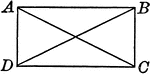### Rectangle With Diagonals

Illustration to show that if the diagonals of a parallelogram are equal, the figure is a rectangle.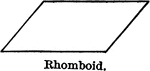### Rhomboid

Illustration of a rhomboid, which is a parallelogram which has its angles oblique angles.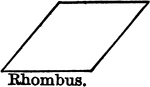### Rhombus

Illustration of a rhombus, which is a rhomboid with all sides equal.### Rhombus

Tangrams, invented by the Chinese, are used to develop geometric thinking and spatial sense. Seven figures…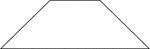### Rhombus

Tangrams, invented by the Chinese, are used to develop geometric thinking and spatial sense. Seven figures…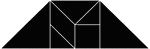### Rhombus

Tangrams, invented by the Chinese, are used to develop geometric thinking and spatial sense. Seven figures…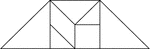### Rhombus

Tangrams, invented by the Chinese, are used to develop geometric thinking and spatial sense. Seven figures…### Right Triangle

Tangrams, invented by the Chinese, are used to develop geometric thinking and spatial sense. Seven figures…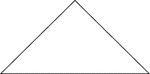### Right Triangle

Tangrams, invented by the Chinese, are used to develop geometric thinking and spatial sense. Seven figures…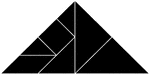### Right Triangle

Tangrams, invented by the Chinese, are used to develop geometric thinking and spatial sense. Seven figures…### Right Triangle

Tangrams, invented by the Chinese, are used to develop geometric thinking and spatial sense. Seven figures…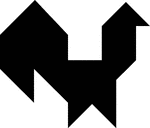### Rooster

Tangrams, invented by the Chinese, are used to develop geometric thinking and spatial sense. Seven figures…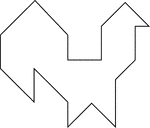### Rooster

Tangrams, invented by the Chinese, are used to develop geometric thinking and spatial sense. Seven figures…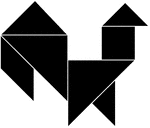### Rooster

Tangrams, invented by the Chinese, are used to develop geometric thinking and spatial sense. Seven figures…